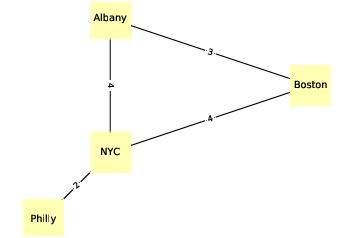# 4.3. NetworkX¶Figure 4.2: An undirected graph that represents driving time between cities.¶

To represent graphs, we’ll use a package called NetworkX, which is the most commonly used network library in Python.

We can create a directed graph by importing NetworkX (usually imported as nx) and instantiating nx.DiGraph:

import networkx as nx
G = nx.DiGraph()


At this point, G is a DiGraph object that contains no nodes and no edges. We can add nodes using the add_node method:

G.add_node('Alice')


Now we can use the nodes method to get a list of nodes:

(G.nodes())
NodeView(('Alice', 'Bob', 'Chuck'))


The nodes method returns a NodeView, which can be used in a for loop or, as in this example, used to make a list.

Adding edges works pretty much the same way:

G.add_edge('Alice', 'Bob')


And we can use edges to get the list of edges:

(G.edges())
[('Alice', 'Bob'), ('Alice', 'Chuck'),
('Bob', 'Alice'), ('Bob', 'Chuck')]


NetworkX provides several functions for drawing graphs; draw_circular arranges the nodes in a circle and connects them with edges:

(nx.draw_circular(G,
node_color=COLORS,
node_size=2000,
with_labels=True)


That’s the code I use to generate Figure 4.1. The option with_labels causes the nodes to be labeled; in the next example we’ll see how to label the edges.

To generate Figure 4.2, I start with a dictionary that maps from each city name to its approximate longitude and latitude:

positions = dict(Albany=(-74, 43),
Boston=(-71, 42),
NYC=(-74, 41),
Philly=(-75, 40))


Since this is an undirected graph, I instantiate nx.Graph:

G = nx.Graph()


Then I can use add_nodes_from to iterate the keys of positions and add them as nodes:

G.add_nodes_from(positions)


Next we will make a dictionary that maps from each edge to the corresponding driving time:

drive_times = {('Albany', 'Boston'): 3,
('Albany', 'NYC'): 4,
('Boston', 'NYC'): 4,
('NYC', 'Philly'): 2}


Now I can use add_edges_from, which iterates the keys of drive_times and adds them as edges:

G.add_edges_from(drive_times)


Instead of draw_circular, which arranges the nodes in a circle, we will use draw, which takes the position dictionary as the second parameter:

nx.draw(G, positions,
node_color=COLORS,
node_shape='s',
node_size=2500,
with_labels=True)


draw uses positions to determine the locations of the nodes.

To add the edge labels, we use draw_networkx_edge_labels:

x.draw_networkx_edge_labels(G, positions,
edge_labels=drive_times)


The edge_labels parameter expects a dictionary that maps from each pair of nodes to a label; in this case, the labels are driving times between cities. And that’s how I generated Figure 4.2.

In both of these examples, the nodes are strings, but in general they can be any hashable type.